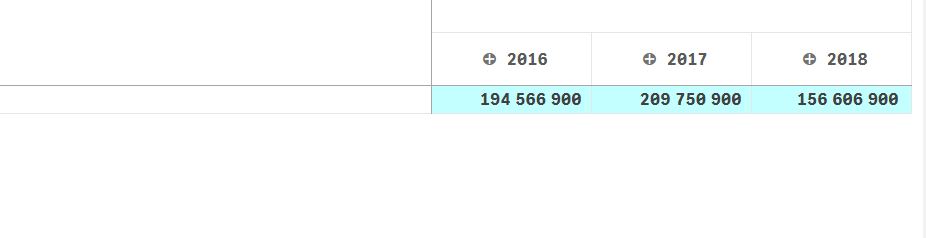New to Qlik Sense

Discussion board where members can get started with Qlik Sense.

Announcements
QlikWorld 2020: Join us May 11 - 14, 2020 in Phoenix, AZ. Register early and save \$400. Learn More
New Contributor

color columns in Qlik sense pivot table (year condition)

Hi Experts,

i need to color my background measure  with this condition :

if(YEAR([DATFCH])=2016,RGB(255,0,0),RGB(196,255,255)),
if(YEAR([DATFCH])=2017,RGB(255,199,0),RGB(196,255,255)),
if(YEAR([DATFCH])=2018,RGB(0,144,255),RGB(196,255,255))

i got the result below : he don't accept the condition (=2016 / 2017/ 2018) as an integer and take the ELSE result (second color)

Any help pleasePartner

Re: color columns in Qlik sense pivot table (year condition)

HI,

Calculate Year in the background i.e. at script level and then use that YEAR field in condition,

If(YEAR='2016', RGB(255,0,0) ,

IF(YEAR='2017',RGB(255,199,0),

IF(YEAR='2018',RGB(0,144,255),RGB(196,255,255)

)))

Thanks,
sasi